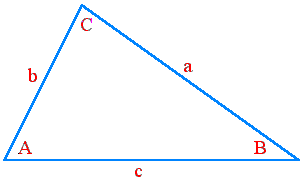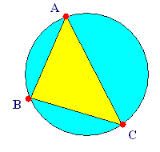Solution of Triangles for IIT JEE

Projection formulas

The solution of triangles is the most important trigonometric problem to solve the triangle or to find out the characteristics of the triangles like measurements of the sides, angles of triangles while having some known values. When we need to find out the angles and all the sides of the triangle using the following rules:

• 1. The law of sines.

• 2. The law of cosines.

• 3. The sum of all the angles of a triangle is always equal to 180 degrees or π radians.

• To solve a particular triangle, multiple cases can occur for e.g., a side is given and angle is to be found out, or sometimes angles are given while the side is to be calculated. The triangle given below is taken as an example to explain some basic rules to find out the solution of triangle, in which angles are denoted in capital letters (A, B and C) and the sides of the triangles are denoted in small letters (a, b and c) while the area of the given triangle is denoted with Δ or S.

Figure 1To find out these cases some of the basic formulas are needed, which gives the relation between the sides of the triangle and the angles of the triangles, which are listed as follows:

• 1. Two angles and one side / One angle and two sides:

• In the above given triangle ABC, if the angle A and angle B is given along with the side b, and we have to calculate the other sides and angles of the triangle. The third remaining angle C can be calculated with the property of a triangle, which includes the sum of three angles which forms the angle of 180 degrees. To find the remaining sides of the triangle, certain fundamental relation between angles and sides are required. In this case, it should be the Sine law.
The law of sine or the sine rule is one of the important laws which gives the relation between the sides and the angles of the triangles. The law of sine states that, the side of the triangle ‘a’ when divided by the sine of an angle ‘A’ is equal to the side of the triangle ‘b’ when divided by the sine of an angle ‘B’ is equal to the side of the triangle ‘c’ when divided by the sine of an angle ‘C’. Mathematically it can be stated as,

Sin A/a = Sin B/b = Sin C/c
Or, a/Sin A = b/Sin B = c/Sin C

So, if two angles are known to us, we can calculate the third angle by knowing that the sum of the three angles of the triangle is 180 degrees and then the sides of the triangles can be calculated with the help of the Sine rule.

• 2. Two sides with one included angle:

• When the two sides of a triangle are given with the angle which is not opposite to the sides given, in this case, the sine law fails and we use cosine law in its place. Firstly the side opposite to the given angle is calculated with the help of the cosine law, and similarly for the sides given we can calculate the angles opposite to them with the help of the Cosine law or if all the sides are known then the remaining angles of the triangles can be calculated with the help of the sine law.

The cosine rule can be stated as when the side of any triangle is given, then the angle opposite to any given side can be calculated and vice-versa i.e.

Cos A = (b2 + c2 – a2) / 2bc
Cos B = (a2 + c2 – b2) / 2ac
Cos C = (a2 + b2 – c2) / 2ab

From the above formulas we can calculate the angles of the triangle with the known sides, while the sides of the triangle can also be calculated with the same formulas which can be written as;

a = √b2 + c2 - 2bcCos A
b = √a2 + c2 – 2acCos B
c = √b2 + a2 – 2baCos C

After finding the sides of the triangle from the above equation, we can calculate the angles of the triangle by rearranging the formulas or by using sine law.

• 3. Two angles and no sides:

• In the above triangle given in Figure 1, when the two angles are known then we can easily calculate the third angle of the triangle by knowing the fact that the total sum of three angles in the triangles combines to form 180 degrees. So, let the angle A and angle B be known in the above shown triangle (fig 1) and angle C is to be calculated, then it can be shown as:
We know that the sum of all the angles of a triangle is 180 degrees. Hence, we get:

∠A + ∠B + ∠C = 180 degrees

∠C = 180 degree – (∠A + ∠B)

Through the above shown equation we can easily calculate the value of the missing third angle of the triangle.

• 4. Three sides and no angles:

• In the above shown figure of triangle ABC, if the three sides (a, b and c) of the triangle are given and no angle is known then we will first try and calculate the angles (A, B and C) with the help of sine law, but the sine law fails to calculate any of the angles in the triangle due to the insufficient data provided. Mathematically,

The Sine rule:-
Sin A/ a = Sin B/ b = Sin C/ c

But the above shown law of sine fails due to the insufficient data provided to find any of the angles.
After that, we will try using cosine rule to find the angles of the given triangle ABC,

The Cosine rule:-
b = √a2 + c2 – 2acCos B …….. (1)

By solving the above equation formed with the help of cosine law we can calculate angle B for the above given triangle.
Squaring on both sides in equation (1) we get,

b2 = a2 + c2 – 2acCos B

By rearranging the above equation and bringing the terms with squares on the same side to get,

2acCos B = a2 +c2 –b2

To find the angle B of the triangle, we have to divide the above figure by 2ac on both sides.

We get
Cos B = (a2 +c2 –b2) / 2ac

Taking the inverse of cosine in the above equation on both the sides, cosine and inverse of cosine get canceled out on the LHS and inverse of cosine is added on the RHS of the above shown equation, we get

B = [Cos-1 (a2 +c2 –b2)] / 2ac ………. (2)

From equation 2 we can calculate the value of angle B as we know all the three sides of the triangle ABC. Similarly, we can calculate another angle with the help of the cosine rule, or we can find one more angle with the help of cosine rule and the third angle can be calculated with the help of the fact that the sum of all the angles of a single triangle is 180 degrees.
From the above shown processes, we can easily calculate the sides and angles of the triangle while any one of that is known and the other is not known. Further, are some of the important formulas to find the area and other important components of the triangle including in solutions of the triangle.

Area of triangle:

Area of the triangle can be calculated as half of the sine of the angle opposite to the two sides known with the length of the two sides, which is denoted by Δ or S. It can be shown as follows,

Area of the triangle (Δ or S) = ½ (bc Sin A)
Or, Δ or S = ½ (ac Sin B)
Or, Δ or S = ½ (ab Sin C)
Area of the triangle can also be calculated as,
Area of the triangle (Δ or S) = √s(s – a)(s – b)(s – c)

Projection formulas:

Some of the projection formulae of the above shown triangle ABC to find out the sides and angles of the triangle can be shown as follows:

• • a = b Cos C + c Cos B

• • b = c Cos A + a Cos C

• • c = a Cos B + b Cos A

•The solution of triangles includes circle inside the triangle or the triangle inscribed inside the circle. When a triangle is inscribed within the circle then the base of the triangle forms the diameter of the circle making it the circum-radius of the triangle which is denoted by R. From the above shown figure of a circle inscribing of a triangle in which AC acts as the diameter of the circle. Its circum-radius can be calculated with the help of the following formula;

R = a/ 2 sin A = b/ 2 sin B = c/ 2 sin C
Where, R is the radius of the circum-circle known as the circum-radius of the triangle.